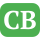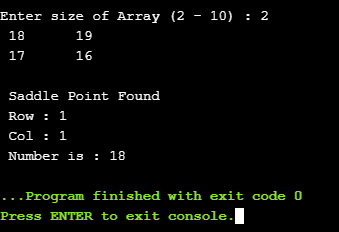CppBuzz.com

# C++ program to find Saddle point in two dimentional array

This program generates random two dimentional array and find Saddle point from the array. User can input size of Array between 2 to 10. Ouput of the program is Row Col and Number.

``````#include <iostream>
using namespace std;

#define MAXROW 10
#define MAXCOL 10

int main() {

srand(time(NULL));

char choice='n';

do{

int no = 0;
no = rand() % 50; //min no can be 1 - 49
int i, j, k, min, max, myMatrix[MAXROW][MAXCOL];
int row, col, flag = 0;
min = max = 0;
int n;

cout<<"\nEnter size of Array (2 - 10) : ";
cin>>n;

if(n<2 && n> 10){
cout<<"\n Error. Size of should be 5 to 10";
return -1;
}

//initialize Array
for(int i=0; i myMatrix[i][j]) {
min = myMatrix[i][j];
col = j;
}
}

//cout<<" \t Min No is " << min;
max = myMatrix[i][col];
for (k = 0; k < n; k++) {
if (max < myMatrix[k][col]) {
max = myMatrix[k][col];
}
}
//cout<<"\t Max no is : " <>choice;

}while(choice=='y');

cout<<"\n Thank you for Using the program";

return 0;
}
```
```

## Finding Saddle point in array